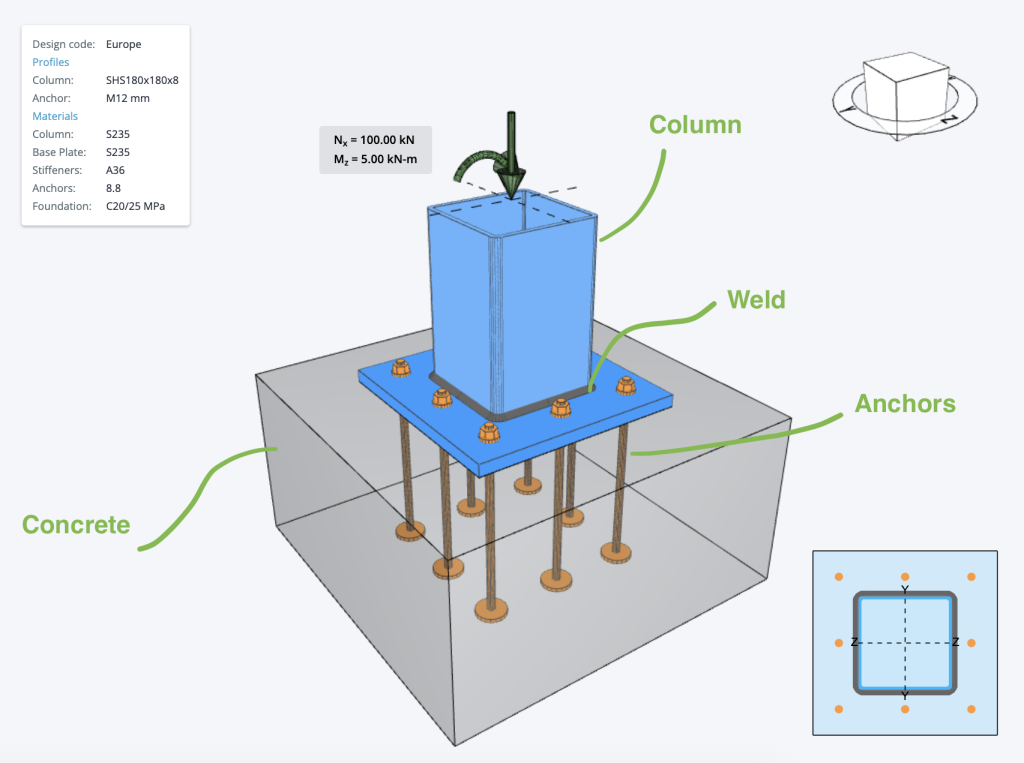SkyCiv Documentation

Your guide to SkyCiv software - tutorials, how-to guides and technical articles

1. Home
2. SkyCiv Connection Design
3. Base Plate Design
4. Steel Base Plate Design Australian Code Example

# Steel Base Plate Design Australian Code Example

Below is an example of some Australian Base Plate Calculations that are commonly used in base plate design. Often when designing base plates, we will consider a few different checks relating to the various components of a base plate, namely:

• The Concrete base – generally checked against bearing and compression forces in reference  to AS3600
• The Welds – welds need to be checked, to ensure they provide adequate restraint and do not fail under stress to AS4100
• Anchor Bolts – can fail due to a number of reasons, as shown below in the example anchor bolt design calculations to AS5216
• Steel Member (Column) checks – usually based on local steel design standardsCurrently, the Steel Base Plate Design module implements the following checks below. The paid version of this software, includes detailed step-by-step calculations, so that engineers can review exactly how these calculations are made!

## Concrete Check

The Steel Base Plate Design checks Concrete Bearing check designed in according to AS3600-2018 and shall satisfy:

$$\phi f_{b} = \phi \times f_{c}^{‘} \times \sqrt{\frac{A_{2}}{A_{1}}} \leq 1.8 \times f_{c}^{‘}$$
where:

• $$\phi$$ – 0.6 – Capacity factor
• $$f_{c}^{‘}$$ – Concrete compressive strength
• $$A_{1}$$ – Base plate area
• $$A_{2}$$ – Concrete (pedestal, foundation) area

## Weld Check

The Steel Base Plate Design checks the weld designed according to AS4100-2021 and shall satisfy:

$$\phi v_{w} = \phi \times 0.6 \times f_{uw} \times t_{t} \leq v_{w}^{*}$$
where:

• $$\phi$$ – 0.80 – Resistance factor for welded connections
• $$f_{uw}$$ – Nominal tensile strength of weld metal
• $$t_{t}$$ – Design throat thickness
• $$v_{w}^{*}$$ – Design force per unit length of weld

## Anchor Bolts Checks

The Steel Base Plate Design check the anchors capacity. Please see below base plate anchor bolt per Australian code:

### Bolt in tension

A bolt subject to design tension force is designed according to AS5216:2018 and shall satisfy:

$$\phi M_{s} N_{tf} = \phi M_{s} \times A_{s} \times f_{uf} \leq N^{*}_{tf}$$
where:

• $$\phi M_{s}$$ – Capacity factor for steel in tension. where $$\frac{5 \times f_{yf} }{ 6 \times f_{uf} } \leq \frac{1}{1.4}$$
• $$A_{s}$$ – tensile stress area
• $$f_{yf}$$ – Minimum yield strength of the bolt
• $$f_{uf}$$ – Minimum tensile strength of the bolt

### Bolt in shear

A bolt subject to a design shear force is designed according to AS5216:2018 and shall satisfy:

$$\phi M_{s} V_{Rk,s} = 0.62 \times \phi M_{s} \times A_{s} \times f_{uf} \leq V^{*}_{f}$$
where:

• $$\phi M_{s}$$ – Capacity factor for steel in tension. where $$\frac{5 \times f_{yf} }{ 6 \times f_{uf} } \leq \frac{1}{1.4}$$
• $$A_{c}$$ – Minor diameter area of the bolts
• $$f_{uf}$$ – Minimum tensile strength of the bolt

### Anchor Breakout

A bolt subject to a design breakout is designed according to AS5216:2018 and shall satisfy:

$$\phi M_{c} N_{Rk,c} = \phi M_{c} \times N_{Rk,c}^{o} \times \frac{A_{c,N}}{A_{c,N}^{o}} \times \phi _{s,N} \times \phi _{re,N} \times \phi _{ec,N} \times \phi _{M,N} \leq N^{*}_{tf,g}$$
where:

• $$\phi M_{c}$$ – Capacity factor for anchor failure modes connected to concrete \)
• $$N_{Rk,c}^{o}$$ – Characteristic strength of a fastener, remote from the effects of adjacent fasteners or edges of the concrete member.
• $$N_{tf,g}^{*}$$ – Sum of tension forces of anchors with common concrete breakout cone area.
• $$A_{c,N}$$ – Concrete breakout cone area for group of anchors.
• $$A_{c,N}^{o}$$ – Concrete breakout cone area for single anchor not influenced by edges.
• $$\phi _{s,N}$$ – Parameter related to the distribution of stresses in the concrete due to the proximity of the fastener to an edge of the concrete membe.
• $$\phi _{re,N}$$ – Parameter accounting for the shell spalling.
• $$\phi _{ec,N}$$ – Modification factor for anchor groups loaded eccentrically in tension.
• $$\phi _{M,N}$$ – Parameter accounting for the eﬀect of a compression force between the ﬁxture and concrete.

### Concrete pryout check

A bolt subject to design tension force is designed according to AS5216:2018 and shall satisfy:

$$\phi M_{c} V_{Rk,cp} = \phi M_{c} \times k_{s} \times N_{Rk,c} \leq V^{*}_{f}$$
where:

• $$\phi M_{c}$$ – Capacity factor for anchor failure modes connected to concrete \)
• $$k_{s}$$ – Parameter published in the Report of Assessment
• $$N_{Rk,c}$$ – Characteristic concrete cone strength for a single fastener or fastener in a group

## Anchor Bolts Utility ratio Checks

### Utility ratio for steel

Interaction of tensile and shear forces of steel

$$\left( \frac{N^{*}}{ \phi M_{c} \times N_{Rk} } \right)^{2} + \left( \frac{V^{*}}{ \phi M_{c} \times V_{Rk} } \right)^{2} \leq 1.0$$

### Utility ratio for concrete

Interaction of tensile and shear forces in concrete

$$\left( \frac{N^{*}}{ \phi M_{c} \times N_{Rk} } \right)^{1.5} + \left( \frac{V^{*}}{ \phi M_{c} \times V_{Rk} } \right)^{1.5} \leq 1.0$$